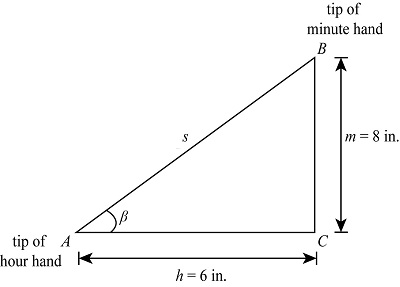# The minute and hour hands on the face of a school clock are 8 inches and 6 inches long,...

## Question:

The minute and hour hands on the face of a school clock are 8 inches and 6 inches long, respectively. Find the rate of change, in inches per minute, of the distance between the tips of the hands at 9:00.

## Calculus:

Calculus is part of mathematics study which helps to analyse the continuous change of the function or data overt the shape and algebra known as calculus. The differential and integration are part of the calculus.

Given Data:

• The length of minute hand is: {eq}m = 8\;{\rm{in}} {/eq}
• The length of hour hand is: {eq}h = 6\;{\rm{in}} {/eq}

The triangle diagram of given problem isThe angle between minute and hour hands is {eq}\beta = {90^o} {/eq} at 9:00 on face of school clock.

The distance between tips of minute and hour hand is: {eq}s {/eq}

The expression by Pythagoras theorem for triangle ABC is

{eq}{s^2} = {m^2} + {h^2} - 2\cos \beta {/eq}

Substitute and solve the above expression

{eq}\begin{align*} {s^2} &= {\left( 8 \right)^2} + {\left( 6 \right)^2} - 2 \times 8 \times 6\cos \beta \\ {s^2} &= 100 - 96\cos \beta \cdots\cdots\rm{(I)} \end{align*} {/eq}

Differentiate the above expression with respect to time

{eq}\begin{align*} \dfrac{{d\left( {{s^2}} \right)}}{{dt}} &= \dfrac{{d\left( {100 - 96\cos \beta } \right)}}{{dt}}\\ 2s\dfrac{{ds}}{{dt}} &= - 96\left( { - \sin \beta } \right)\left( {\dfrac{{d\beta }}{{dt}}} \right)\\ s\dfrac{{ds}}{{dt}} &= 48\left( {\sin \beta } \right)\left( {\dfrac{{d\beta }}{{dt}}} \right) \cdots\cdots\rm{(II)} \end{align*} {/eq}

Here {eq}\dfrac{{d\beta }}{{dt}} {/eq} is angular speeds which try to meet hour hand by minute hand

The minute hand moves one complete rotation per 3600 second and hour hand complete 1 degree per 120 second when minute hand complete one rotation

The expression for rate of minute hand is

{eq}\begin{align*} \dfrac{{dm}}{{dt}} &= \dfrac{{{{360}^o}}}{{3600}}\\ &= \dfrac{1}{{10}} \end{align*} {/eq}

The expression for rate of hour hand is

{eq}\dfrac{{dh}}{{dt}} = \dfrac{{{1^o}}}{{120}} {/eq}

The expression for angular speeds which try to meet hour hand by minute hand

{eq}\dfrac{{d\beta }}{{dt}} = \dfrac{{dm}}{{dt}} - \dfrac{{dh}}{{dt}} {/eq}

Substitute and solve the above expression

{eq}\begin{align*} \dfrac{{d\beta }}{{dt}} &= \dfrac{1}{{10}} - \dfrac{1}{{120}}\\ &= \dfrac{{12 - 1}}{{120}}\\ &= \dfrac{{11}}{{120}} \end{align*} {/eq}

Substitute and solve the expression (I)

{eq}\begin{align*} {s^2} &= {\left( 8 \right)^2} + {\left( 6 \right)^2} - 2 \times 8 \times 6\cos \left( {{{90}^o}} \right)\\ {s^2} &= 100 - 96\cos \left( {{{90}^o}} \right)\\ s &= 10 \end{align*} {/eq}

Substitute and solve the expression (II)

{eq}\begin{align*} \left( {10} \right)\dfrac{{ds}}{{dt}} &= 48\left( {\sin \left( {{{90}^o}} \right)} \right)\left( {\dfrac{{11}}{{120}}} \right)\\ \dfrac{{ds}}{{dt}} &= 0.0440\;{\rm{in/s}} \end{align*} {/eq}

Thus the rate of change of distance from tip of minute and hour hand is {eq}0.0440\;{\rm{in/s}} {/eq}